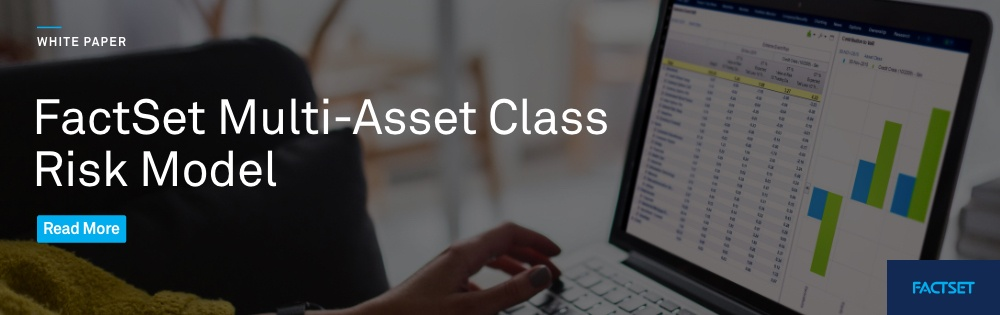English# The Growing Necessity for Multi-Asset Class Risk Factor Models

###### Risk, Performance, and Reporting

By FactSet Insight  |  September 28, 2020

A growing number of investment managers today regard the function of the enterprise risk management system as moving above and beyond simple compliance into the heart of the investment process. Risk models are no longer simply used to monitor risk, but to drive portfolio performance. They are used for risk forecasting (as forward-looking tools to predict future volatility), risk analysis (as tools to understand current risk exposures), and risk attribution (as guides to past performance, evaluating actual returns in relation to risk exposures of the portfolio).

A typical portfolio contains hundreds or thousands of securities and quantifying the risks of each portfolio holding and their complex interplay can be quite a daunting task. Factor risk models provide portfolio managers with a convenient way of reducing this complexity to a manageable set of precise market risk exposures. They supply a detailed understanding of the sources of risk in each portfolio, help portfolio managers decompose risk into contributions from well-defined market factors, and quantify correlations between these contributions. Factor risk models are indispensable for understanding the contributions of different portfolio components to total risk and for measuring and controlling portfolio risk exposures.

A typical factor risk model represents the return of every security in the market as a function of a limited, predefined set of factors.

A typical factor risk model represents the return of every security in the market as a function of a limited, predefined set of factors. It could be a simple linear function, in which case we have a linear model that is simple to implement but that does not necessarily capture the full behavior of complex financial instruments, especially in the tails of the return distribution. Alternatively, the model may compute the return of each instrument as a nonlinear function of the factors. For example, when the model is based on full and exact repricing of the instruments or a nonlinear interaction between factors and sources of valuation as in the case of structural spread models. Such nonlinear models will be more difficult to implement and will require more computational power to run, but they will provide more precise estimates of tail and complex derivatives risk.

In both cases, the risk factor model estimates the risk characteristics of the portfolio using a covariance matrix of factor returns. For most portfolios, the number of factors in the model is much smaller than the number of securities in the portfolio. Thus, the factor covariance matrix requires fewer parameters to estimate, leading to a much more stable and robust estimation of portfolio risks. Moreover, the factors can be designed in such a way as to increase the stability of the covariance matrix and the quality of the risk estimators. The factors of the model are designed on one hand to capture systematic market fluctuation and on the other hand, to be able to reveal a detailed structure of the market exposures of each portfolio. For example, a single interest rate factor would capture the general movement of the interest rate curve but not the details of the portfolio exposures to the back and front ends of the curve or the slope of the curve. In this case, it is evident that more interest rate risk factors are required to quantify the portfolio exposure to these risks. At the same time, the total number of interest rate factors should remain limited to keep the dimensionality of the problem under control. Thus, factor risk model construction is, among other things, an exercise in the optimal choice of the set of risk factors.

Multi-asset class risk factor models are becoming necessary now, as more and more investment managers are operating on global, multi-asset type markets.

Multi-asset class risk factor models are especially difficult because of the complexity of calculating risks across different asset types. However, they are becoming necessary now, as more and more investment managers are operating on global, multi-asset type markets. Historically, the risks of such portfolios have been estimated by independently modeling risks of holdings of each asset type, like equities or fixed income, and then aggregating those risks into a multi-asset class portfolio risk. This can result in significant errors in estimated risk because this methodology does not take into account correlations between different asset classes. A multi-asset class risk factor model that is based on a set of factors that capture systematic shifts in all markets (equities, fixed income, commodities, etc.) remedies this deficiency and allows the portfolio manager to accurately estimate portfolio risk and its components stemming from different asset type markets.

Download our latest white paper to review the structure of the FactSet multi-asset class (MAC) factor risk framework and the types of factors used in its models, and learn about the estimation techniques used to compute risk factors and the corresponding security returns.